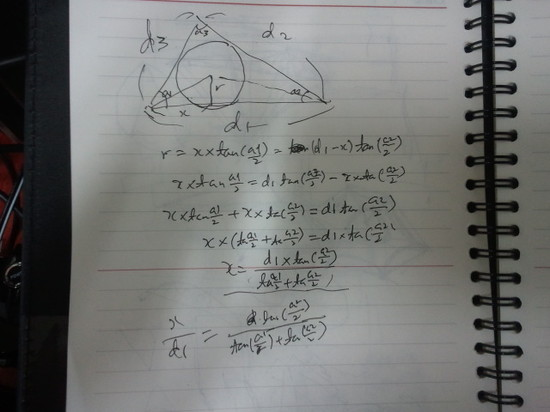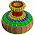## Sunday, August 25, 2013

### 3-points Circle, 3-points archDraw in Autacad with 6 CirclesDraw in Revit Only One Circle?
There are various ways to draw circles in Autocad, and they are very useful.

But, there's nothing like that in Revit. Center point-Radius, this is the only way.

So, here is a question. Is it possible to make circle with 2-points or 3-points method in Revit just like in Autocad? To tell the conclusion first, yes it is.

I don't think this 2-points method is very useful, but it must be much easier to understand others after get this. Acually, I developed 3points way first.Adaptive components were used in every ways.
1. Draw a line with adaptive points at the both ends
2. Place a point at the middle of the line
3. Draw a circle that has it's center point at the drawn point.
4. Give lables and connect them with formulas.
And here is a making video.

### 3-Points Circle

Developing 3-Points Circle is even more complicated. Because a center point  can be anywhere depends on the positions of three points.

#### 1st Approach - Using Angles

First of all, Pattern Based templates that has adaptive reference lines must be used for using angle parameters as reporting ones and make available using in formulas. If not, this error message shows up.

Before make this, I had to analyse the relation between a triangle and a circumscribed circle of it.

From this, I found that two more points(P4,P5) and one angle(a3) are necessary except adaptive ones.
P1,P2,P3 and a1, a2 and d can be get as reporting parameter from adaptive points and lines.

P4 will be always at the middle of the line P1-P2, and P5 can be located from P4 if we can get h.
h and r can be found if we have a3 and d/2 using trigonometric function.

With some formulas that I learned centuries ago, I could get this formula.

a3=a1+a2-90˚

And d/2? It's already given. Now h and r can be found like this.

h=(d/2)*tan(a3)
r=(d/2)/cos(a3)

And this worked!

#### 2nd Approach - Using Distances

Angle method that I explained above is not very difficult, but if angles can not be used as reporting parameters that can be used in formulas?  Reference lines can be host references only in Pattern Based templates.

I tried to make another way without using angles for reporting parameters in a Genetic Adaptive template. Only distances between three adaptive points were used for reporting parameters.

To get the location of center points, angles are always necessary. I just didn't use angels but distances as reporting parameters to get what I needed.
After analysation, I found it that area of a triangle is necessary to get angles of it. And areas can be found with distances using 'Heron's formula'.

when s= d1+d2+d3
area=sqrt((s*(s-d1)*(s-d2)*(s-d3))

This is it. And from this formulas I could get angles using trigonometric.

when x=area*2/d1
a1=asin(x/d2)
a2=asin(x/d3)

Rest of necessary numbers can be found just like Angle-Method that I explained at the 1st approach.

But, after I made this in Revit, I found there is a little faulty in this formulas.
If distance d1 is not the longest one, a circle goes the wrong way. It's because a degree over 90˚ can't be found with these formulas.
I could fix this with <if> formula like this. (used Pythagorean theorem to recognize 90˚)

a1=if(d1^2+d2^2>d3^2,asin(x/d2),180˚-asin(x/d2))
a2=if(d1^2+d3^2>d2^2,asin(x/d3),180˚-asin(x/d3))

Finally, the family could make circle only with distances between three points.

#### 3rd Approach - Inscribed Circle

After I got circumscribed circle, making inscribed circle was much easier because I've got lots of numbers already. Only one page of my note was all I need.(I know I have bad hand writing. I rarely use this)

I could make this with some more numbers.

Let's see how this works.

### What for is this necessary?

It's not necessary at all. But this can make it easier to make some adaptive families.

I used 3Points-Circle to make a 3Points-Arch based family. I'm sure you can make more useful ones with it.

Enjoy.  :-)

1.Hi. I enjoyed reading your post, as I was working on modeling circumscribed triangles to lead to creation of a Delaunay triangulation of a surface. Your work inspired me to develop a largely graphical approach to finding the circumcenter, which I have blogged:
http://texasarchitecture.blogspot.com/2013/09/circumscribed-triangles-helpers-for.html
Thank you for the clever modeling. Best wishes.

1.2.I tried to open the curved frame in Revit 2013. Do I need Revit 2014 to open it?

1.Unfortunately, yes.Sorry.

3.Gracias por tu aporte. Un articulo verdaderamente iluminante. Gracias tambien por tus "descargables"

1.Gracias por la lectura y sus amables palabras.

2.gracias por compartir tus conocimientos

4.it seem that the adaptive option only works in 2D.
have you tried to make it work in 3D (out of plane)?
the issue is that the reporting parameter for the edges can not be based on the triangle of the 3 points, but the x/y plane.

1.Right. I ignored that part to make the story simple. It can be achieved with little more modification. Or use the other version which used intersect point.
5.